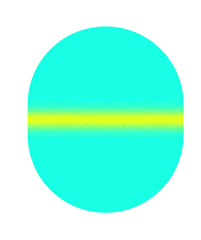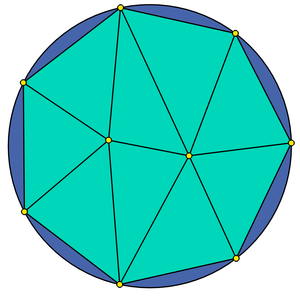# Project A2 • Numerical methods for wave problems with nontrivial boundary conditions

### Principal investigators

 Prof. Dr. Marlis Hochbruck (7/2015 - ) Prof. Dr. Christian Lubich (7/2015 - )

### Project summary

Boundary conditions are an essential part of mathematical models of wave phenomena that are confined in a bounded spatial domain and describe the behavior of the waves at the boundary.
During the rise of computing power and demand for simulation, nontrivial boundary conditions became increasingly important, for example to effectively model complex surface phenomena or as artificial boundary conditions imposed on domains truncated for computational purposes. Often, such nontrivial boundary conditions are not covered by standard numerical analysis.

The aim of this project is the construction of stable numerical schemes and to provide a rigorous error analysis for wave equations with two types of nontrivial boundary conditions: First, transparent boundary conditions that allow to truncate an unbounded domain to a bounded non-convex domain suitable for numerical approximation. Second, dynamic boundary conditions which are used in sophisticated models for surface phenomena.

## Transparent boundary conditions

Transparent boundary conditions are often used in the numerical approximation of models for wave-type phenomena on unbounded domains, where the initial data and the source is contained in a bounded domain. Many efficient techniques (perfectly matched layers, fast multipole methods, etc.) are known for convex domains. This subproject is concerned with the case of non-convex domains. A particular difficulty is the fact that waves can leave and reenter the domain.
We devised stable and convergent numerical methods which only involve the interior of this domain and its boundary. The transparent boundary conditions are imposed as a time-dependent boundary integral equation, in which the appearing Calderon operator is non-local in both space and time.

Particular difficulties of the analysis lay in finding the suitable functional analytic setting which permits the right definition of boundary integral operators, proving the coercivity of the Calderon operator, and ensuring stability of the numerical methods.

## Research and results

• Maxwell's equations [KL17]: The suitable abstract framework, allowing a boundary integral formulation, is developed. We show the coercivity of the Calderon operator, upon which the stability of the scheme relies. We prove stability and convergence of a numerical method, using a discontinuous Galerkin method (cf. [HS19] from A4) and leapfrog scheme in the interior coupled to boundary elements and (BDF2) convolution quadrature on the boundary.
• Elastodynamics [Ebe18]: Again the key issue is finding the coercivity of the Calderon operator encoding the transparent boundary conditions, and the abstract framework, which allows to prove its coercitvity. Stability and convergence of a numerical scheme, coupling finite elements and leapfrog time-stepping in the interior with boundary elements and (BDF2) convolution quadratures on the boundary, is proved. Numerical experiments and the handling of implementation issues involving the hyper-singular operator are presented in [Ebe19].
• Runge—Kutta coercivity [BL19]: The coercivity property plays a central role in showing stability of the interor—exteror coupling. Convolution quadratures based on A-stable multistep methods are known to preserve this property. In this paper we study the question: “which Runge—Kutta-based convolution quadratures inherit this property?” It is shown that this holds without any restriction for the third-order Radau IIA method, and on permitting a shift in the Laplace domain variable, this holds for all algebraically stable Runge–Kutta methods and hence for methods of arbitrary order. The results are illustrated with various numerical experiments.

## Dynamic boundary conditions

In contrast to Dirichlet or Neumann boundary conditions, dynamic boundary conditions do not neglect the momentum of the wave on the boundary. They are particularly suited to model flexible boundaries like an eardrum and often appear as effective models for wave propagation in in thin layers. Hence, the simulation of these kind of boundary conditions is important in many applications.The animations show the results of three numerical simulations with different boundary conditions. On the left, we have homogeneous Neumann boundary conditions. In the middle and on the right, the animations show simulations with dynamic boundary conditions. Note that for kinetic boundary conditions (middle) there are small waves that stick at the boundary. Acoustic boundary conditions (right) introduce a second variable at the boundary which is visualized by black arrows.

A particular difficulty for the space discretization are domains with curved boundaries which naturally appear for certain dynamic boundary conditions. Only few numerical methods can treat such domains exactly. Instead, most numerical methods are applied on an approximated domain. This makes the implementation as well as the error analysis more involved.The picture show two meshs of the blue disc. On the left, we have a triangulation that approximates the disc very crudely. The right picture shows the corresponding isoparametric triangulation with quadratic elements that cover the disc much better.

Despite the fact that the error analysis of such problems mostly use the same ideas, it is usually carried out for a particular combination of wave equation and numerical method. To avoid repetitive work and ease the derivation of new results, we developed the unified error analysis which applies to many different kinds of wave equations and numerical methods.

## Research and results

• Unified error analysis of non-conforming space discretizations for wave-type equations: We propose an abstract framework for non-conforming space discretizations of linear and semi-linear wave equations, which combines a variational approach with semigroup theory. The error estimates we derive in this framework allow applicants to easily evaluate the accuracy of a method for their concrete problem by inserting basic information about the numerical method. [DHS19, Hip17, Lei17]. Our unified error analysis is also used in other projects, e.g. to analyze the error of a heterogeneous multiscale method for Maxwell’s equation [Bernhards MA]. Our future aim is to further extend this ansatz to cover nonlinear cases.
• Energy norm error estimates for space discretizations: We consider isoparamteric finite element approximations. Using the unified error analysis, we prove convergence rates in an energy norm for the wave equation with acoustic boundary conditions in [DHS19] and kinetic boundary conditions in [Hip17]. Moreover, we provide a rigorous error analysis of the wave equation with semi-linear kinetic boundary conditions. [Lei17]
• $L^2$ error estimates for space discretizations of wave equations require more direct techniques then the ones available via the unified error analysis (where we derive estimates in the energy norm). Hence the final convergence results themselves are less 'unified' [HK18]. We show semi-discrete convergence estimates in $L^2$ norm, using non-conforming finite elements for four representative examples of wave equations with dynamic boundary conditions, working in the unified setting as long as the analysis allows. Fully discrete $L^2$ norm convergence results, using algebraically stable and coercive Runge-Kutta methods, are also shown.
• Efficient time integration: We are particularly interested in time integration schemes that exploit the structure of the corresponding problems. Such methods can be much more efficient than traditional implicit methods as e.g. the Crank-Nicolson method while having superior stability properties compared to explicit methods as the Leapfrog scheme. We have first results for semi-linear problems which show the second-order convergence of a linear implicit method, which does not require the solution of nonlinear systems of equations [in preparation].
• Full discretization: The framework of the unified error analysis also allows to analyze fully discrete approximations obtained by the method of lines. As examples, we consider the Crank-Nicolson method [Hip17] and algebraically stable and coercive Runge-Kutta methods [BL19] and show that the full discretization error is bounded by the sum of the (stiff) time integration error and the space discretization error.

## Publications

1. and . Runge–Kutta convolution coercivity and its use for time-dependent boundary integral equations. IMA J. Numer. Anal., 39(3):1134–1157, July . [preprint] [bibtex]

2. , , and . Unified error analysis for nonconforming space discretizations of wave-type equations. IMA J. Numer. Anal., 39(3):1206–1245, July . [preprint] [bibtex]

3. , , , and . Analytical and numerical analysis of linear and nonlinear properties of an rf-SQUID based metasurface. Phys. Rev. B, 99(7):075401, February . [preprint] [bibtex]

4. and . Stability and convergence of time discretizations of quasi-linear evolution equations of Kato type. Numer. Math., 138(2):365–388, February . [preprint] [bibtex]

5. and . Stable and convergent fully discrete interior-exterior coupling of Maxwell's equations. Numer. Math., 137(1):91–117, September . [preprint] [bibtex]

6. and . Numerical analysis of parabolic problems with dynamic boundary conditions. IMA J. Numer. Anal., 37(1):1–39, January . [preprint] [bibtex]

## Preprints

1. and . Finite element discretization of semilinear acoustic wave equations with kinetic boundary conditions. CRC 1173 Preprint 2019/26, Karlsruhe Institute of Technology, December . [bibtex]

2. and . Finite element error analysis of wave equations with dynamic boundary conditions: $L^2$ estimates. CRC 1173 Preprint 2018/53, Karlsruhe Institute of Technology, December . Revised version from January 2020. [bibtex]

## Theses

1. . Semilineare Wellengleichungen mit dynamischen Randbedingungen. Master's thesis, Karlsruhe Institute of Technology (KIT), September . [bibtex]

2. . A unified error analysis for spatial discretizations of wave-type equations with applications to dynamic boundary conditions. PhD thesis, Karlsruhe Institute of Technology (KIT), June . [bibtex]

## Other references

1. . An implementation and numerical experiments of the FEM‐BEM coupling for the elastodynamic wave equation in 3D. ZAMM Z. Angew. Math. Mech., 99(12):e201900050, December . [bibtex]

2. and . Upwind discontinuous Galerkin space discretization and locally implicit time integration for linear Maxwell's equations. Math. Comp., 88(317):1121–1153, May . [preprint] [bibtex]

3. . The elastic wave equation and the stable numerical coupling of its interior and exterior problems. ZAMM Z. Angew. Math. Mech., 98(7):1261–1283, July . [bibtex]

Project-specific staff
NamePhoneE-Mail+49 721 608-42771 volker grimmKcd2∂kit edu
+49 721 608-42060 marlis hochbruckDme9∂kit edu
+49 7071 29-72934 kovacsVus3∂na uni-tuebingen de
+49 721 608-47746 jan leiboldEdp7∂kit edu
+49 7071 29-72935 lubichFvg8∂na uni-tuebingen de
+49 7071 29-72932 nickNzf8∂na uni-tuebingen de
+49 721 608-44342 stefan schrammerTnd5∂kit edu

Former staff
NameTitleFunctionDr. Postdoctoral researcher
Dr. Postdoctoral researcher